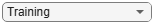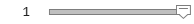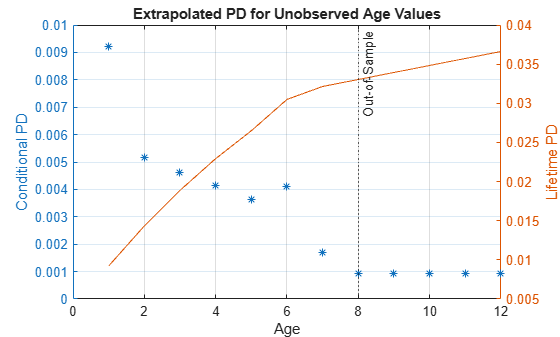# predict

Compute conditional PD

## Syntax

``conditionalPD = predict(pdModel,data)``

## Description

example

````conditionalPD = predict(pdModel,data)` computes the conditional probability of default (PD). ```

## Examples

collapse all

This example shows how to use `fitLifetimePDModel` to fit data with a `Probit` model and then predict the conditional probability of default (PD).

```load RetailCreditPanelData.mat disp(head(data))```
``` ID ScoreGroup YOB Default Year __ __________ ___ _______ ____ 1 Low Risk 1 0 1997 1 Low Risk 2 0 1998 1 Low Risk 3 0 1999 1 Low Risk 4 0 2000 1 Low Risk 5 0 2001 1 Low Risk 6 0 2002 1 Low Risk 7 0 2003 1 Low Risk 8 0 2004 ```
`disp(head(dataMacro))`
``` Year GDP Market ____ _____ ______ 1997 2.72 7.61 1998 3.57 26.24 1999 2.86 18.1 2000 2.43 3.19 2001 1.26 -10.51 2002 -0.59 -22.95 2003 0.63 2.78 2004 1.85 9.48 ```

Join the two data components into a single data set.

```data = join(data,dataMacro); disp(head(data))```
``` ID ScoreGroup YOB Default Year GDP Market __ __________ ___ _______ ____ _____ ______ 1 Low Risk 1 0 1997 2.72 7.61 1 Low Risk 2 0 1998 3.57 26.24 1 Low Risk 3 0 1999 2.86 18.1 1 Low Risk 4 0 2000 2.43 3.19 1 Low Risk 5 0 2001 1.26 -10.51 1 Low Risk 6 0 2002 -0.59 -22.95 1 Low Risk 7 0 2003 0.63 2.78 1 Low Risk 8 0 2004 1.85 9.48 ```

Partition Data

Separate the data into training and test partitions.

```nIDs = max(data.ID); uniqueIDs = unique(data.ID); rng('default'); % for reproducibility c = cvpartition(nIDs,'HoldOut',0.4); TrainIDInd = training(c); TestIDInd = test(c); TrainDataInd = ismember(data.ID,uniqueIDs(TrainIDInd)); TestDataInd = ismember(data.ID,uniqueIDs(TestIDInd));```

Create a `Probit` Lifetime PD Model

Use `fitLifetimePDModel` to create a `Probit` model.

```pdModel = fitLifetimePDModel(data(TrainDataInd,:),"Probit",... 'AgeVar','YOB',... 'IDVar','ID',... 'LoanVars','ScoreGroup',... 'MacroVars',{'GDP','Market'},... 'ResponseVar','Default'); disp(pdModel)```
``` Probit with properties: ModelID: "Probit" Description: "" Model: [1x1 classreg.regr.CompactGeneralizedLinearModel] IDVar: "ID" AgeVar: "YOB" LoanVars: "ScoreGroup" MacroVars: ["GDP" "Market"] ResponseVar: "Default" ```

Display the underlying model.

`disp(pdModel.Model)`
```Compact generalized linear regression model: probit(Default) ~ 1 + ScoreGroup + YOB + GDP + Market Distribution = Binomial Estimated Coefficients: Estimate SE tStat pValue __________ _________ _______ ___________ (Intercept) -1.6267 0.03811 -42.685 0 ScoreGroup_Medium Risk -0.26542 0.01419 -18.704 4.5503e-78 ScoreGroup_Low Risk -0.46794 0.016364 -28.595 7.775e-180 YOB -0.11421 0.0049724 -22.969 9.6208e-117 GDP -0.041537 0.014807 -2.8052 0.0050291 Market -0.0029609 0.0010618 -2.7885 0.0052954 388097 observations, 388091 error degrees of freedom Dispersion: 1 Chi^2-statistic vs. constant model: 1.85e+03, p-value = 0 ```

Predict on Training and Test Data

Predict the PD for training or test data sets.

```DataSetChoice ="Training"; if DataSetChoice=="Training" Ind = TrainDataInd; else Ind = TestDataInd; end % Predict conditional PD PD = predict(pdModel,data(Ind,:)); head(data(Ind,:))```
```ans=8×7 table ID ScoreGroup YOB Default Year GDP Market __ __________ ___ _______ ____ _____ ______ 1 Low Risk 1 0 1997 2.72 7.61 1 Low Risk 2 0 1998 3.57 26.24 1 Low Risk 3 0 1999 2.86 18.1 1 Low Risk 4 0 2000 2.43 3.19 1 Low Risk 5 0 2001 1.26 -10.51 1 Low Risk 6 0 2002 -0.59 -22.95 1 Low Risk 7 0 2003 0.63 2.78 1 Low Risk 8 0 2004 1.85 9.48 ```
`disp(PD(1:8))`
``` 0.0095 0.0054 0.0045 0.0039 0.0036 0.0036 0.0017 0.0009 ```

You can analyze and validate these predictions using `modelDiscrimination` and `modelAccuracy`.

This example shows how to use `fitLifetimePDModel` to fit data with a `Cox` model and then predict the conditional probability of default (PD).

```load RetailCreditPanelData.mat disp(head(data))```
``` ID ScoreGroup YOB Default Year __ __________ ___ _______ ____ 1 Low Risk 1 0 1997 1 Low Risk 2 0 1998 1 Low Risk 3 0 1999 1 Low Risk 4 0 2000 1 Low Risk 5 0 2001 1 Low Risk 6 0 2002 1 Low Risk 7 0 2003 1 Low Risk 8 0 2004 ```
`disp(head(dataMacro))`
``` Year GDP Market ____ _____ ______ 1997 2.72 7.61 1998 3.57 26.24 1999 2.86 18.1 2000 2.43 3.19 2001 1.26 -10.51 2002 -0.59 -22.95 2003 0.63 2.78 2004 1.85 9.48 ```

Join the two data components into a single data set.

```data = join(data,dataMacro); disp(head(data))```
``` ID ScoreGroup YOB Default Year GDP Market __ __________ ___ _______ ____ _____ ______ 1 Low Risk 1 0 1997 2.72 7.61 1 Low Risk 2 0 1998 3.57 26.24 1 Low Risk 3 0 1999 2.86 18.1 1 Low Risk 4 0 2000 2.43 3.19 1 Low Risk 5 0 2001 1.26 -10.51 1 Low Risk 6 0 2002 -0.59 -22.95 1 Low Risk 7 0 2003 0.63 2.78 1 Low Risk 8 0 2004 1.85 9.48 ```

Partition Data

Separate the data into training and test partitions.

```nIDs = max(data.ID); uniqueIDs = unique(data.ID); rng('default'); % for reproducibility c = cvpartition(nIDs,'HoldOut',0.4); TrainIDInd = training(c); TestIDInd = test(c); TrainDataInd = ismember(data.ID,uniqueIDs(TrainIDInd)); TestDataInd = ismember(data.ID,uniqueIDs(TestIDInd));```

Create a `Cox` Lifetime PD Model

Use `fitLifetimePDModel` to create a `Cox` model.

```ModelType ="cox"; pdModel = fitLifetimePDModel(data(TrainDataInd,:),ModelType,... 'IDVar','ID','AgeVar','YOB',... 'LoanVars','ScoreGroup','MacroVars',{'GDP' 'Market'},... 'ResponseVar','Default'); disp(pdModel)```
``` Cox with properties: TimeInterval: 1 ExtrapolationFactor: 1 ModelID: "Cox" Description: "" Model: [1x1 CoxModel] IDVar: "ID" AgeVar: "YOB" LoanVars: "ScoreGroup" MacroVars: ["GDP" "Market"] ResponseVar: "Default" ```

Display the underlying model.

`disp(pdModel.Model)`
```Cox Proportional Hazards regression model: Beta SE zStat pValue __________ _________ _______ ___________ ScoreGroup_Medium Risk -0.6794 0.037029 -18.348 3.4442e-75 ScoreGroup_Low Risk -1.2442 0.045244 -27.501 1.7116e-166 GDP -0.084533 0.043687 -1.935 0.052995 Market -0.0084411 0.0032221 -2.6198 0.0087991 ```

Predict on Age Values not Observed in the Training Data

Cox models make predictions for the range of age values observed in the training data. To extrapolate for ages larger than the maximum age in the training data, an extrapolation rule is needed.

When using `predict` with a `Cox` model, you can set the `ExtrapolationFactor` property of the `Cox` model. By default, the `ExtrapolationFactor` is set to `1`. For age values (`AgeVar`) greater than the maximum age observed in the training data, `predict` computes the conditional PD using the maximum age observed in the training data. In particular, the predicted PD value is constant if the predictor values do not change and only the age values change when the `ExtrapolationFactor` is `1`.

To illustrate this, select the rows corresponding to a single ID and add new rows with new, incremental age values beyond the maximum observed age in the training data. The maximum age observed in the training data is 8; for illustration purposes, add rows with ages `9`, `10`, `11`, and `12`.

```% Select rows corresponding to one ID % ID 1 goes from row 1 through 8 % Only the ID, Age (YOB) and predictor variables are needed dataNewAge = data(1:8,{'ID' 'YOB' 'ScoreGroup' 'GDP' 'Market'}); % Allocate more rows % This line copies the same predictor values going forward dataNewAge(9:12,:) = repmat(dataNewAge(8,:),4,1); % Reset age values to 9, 10, 11, 12 dataNewAge.YOB(9:12) = (9:12)'; % Show the new dataset disp(dataNewAge)```
``` ID YOB ScoreGroup GDP Market __ ___ __________ _____ ______ 1 1 Low Risk 2.72 7.61 1 2 Low Risk 3.57 26.24 1 3 Low Risk 2.86 18.1 1 4 Low Risk 2.43 3.19 1 5 Low Risk 1.26 -10.51 1 6 Low Risk -0.59 -22.95 1 7 Low Risk 0.63 2.78 1 8 Low Risk 1.85 9.48 1 9 Low Risk 1.85 9.48 1 10 Low Risk 1.85 9.48 1 11 Low Risk 1.85 9.48 1 12 Low Risk 1.85 9.48 ```

When the predictor values are constant in the rows with new age values and the extrapolation factor is `1`, the predicted PD values are constant. If the extrapolation factor is set to a value smaller than `1`, then the predicted PD values decrease more and more for larger age values and decrease towards zero exponentially.

```% Extrapolation factor can be adjusted pdModel.ExtrapolationFactor =1; % Store predicted conditional PD in the same table dataNewAge.PD = predict(pdModel,dataNewAge); disp(dataNewAge)```
``` ID YOB ScoreGroup GDP Market PD __ ___ __________ _____ ______ __________ 1 1 Low Risk 2.72 7.61 0.0092197 1 2 Low Risk 3.57 26.24 0.005158 1 3 Low Risk 2.86 18.1 0.0046079 1 4 Low Risk 2.43 3.19 0.0041351 1 5 Low Risk 1.26 -10.51 0.003645 1 6 Low Risk -0.59 -22.95 0.0041128 1 7 Low Risk 0.63 2.78 0.0017034 1 8 Low Risk 1.85 9.48 0.00092551 1 9 Low Risk 1.85 9.48 0.00092551 1 10 Low Risk 1.85 9.48 0.00092551 1 11 Low Risk 1.85 9.48 0.00092551 1 12 Low Risk 1.85 9.48 0.00092551 ```

Also, it is useful to see the effect of the extrapolation factor on the lifetime prediction.

Plot the predicted conditional PD values and the lifetime PD values to see the effect of the extrapolation factor on both probabilities. The vertical dotted line separates the known age values (up to, and including, the age value `8`), from the age values not observed in the training data (anything greater than `8`). If the extrapolation factor is `1`, the lifetime PD has a steady upward trend and the conditional PDs are constant. If the extrapolation factor is set to a smaller value like `0.5`, the lifetime PD flattens quickly, as the conditional PD quickly drops towards zero.

```dataNewAge.LifetimePD = predictLifetime(pdModel,dataNewAge); figure; yyaxis left plot(dataNewAge.YOB,dataNewAge.PD,'*') ylabel('Conditional PD') yyaxis right plot(dataNewAge.YOB,dataNewAge.LifetimePD) ylabel('Lifetime PD') title('Extrapolated PD for Unobserved Age Values') xlabel('Age') xline(8,':','Out-of-Sample') grid on```## Input Arguments

collapse all

Probability of default model, specified as a previously created `Logistic`, `Probit`, or `Cox` object using `fitLifetimePDModel`.

Data Types: `object`

Data, specified as a `NumRows`-by-`NumCols` table with projected predictor values to make lifetime predictions. The predictor names and data types must be consistent with the underlying model.

Data Types: `table`

## Output Arguments

collapse all

Predicted conditional probability of default values, returned as a `NumRows`-by-`1` numeric vector.

collapse all

### Conditional PD

Conditional PD is the probability of defaulting, given no default yet.

For example, the predicted conditional PD for the second year is the probability that the borrower defaults in the second year, given that the borrower did not default in the first year.

The formula for conditional PD is

`$PD\left(t\right)=P\left\{t-\Delta tt-\Delta t\right\}$`

where

• T is the time to default.

• Δt is the “time interval” consistent with the periodicity of the panel training `data` (for example, one row per year) and the definition of the default indicator values.

The default indicator is `1` if there is a default over a 1-year period. For more information on time intervals, see Time Interval for Logistic Models, Time Interval for Probit Models, and Time Interval for Cox Models.

In the formulas that follow for `Logistic`, `Probit`, and `Cox` models, the notation is:

• X(t) is the predictor data for the row corresponding to time t.

• β is the vector of coefficients of the underlying model.

For `Logistic` models, the conditional PD is computed as:

`$P{D}_{cond}\left(t\right)=\frac{1}{1+\mathrm{exp}\left(-X\left(t\right)\beta \right)}$`

For `Probit` models, the conditional PD is computed as:

`$P{D}_{cond}\left(t\right)=\varphi \left(X\left(t\right)\beta \right)$`

For `Cox` models, the conditional PD is computed as

`$P{D}_{cond}\left(t\right)=1-\frac{S\left(t\right)}{S\left(t-\Delta t\right)}$`

where S is the survival function. The survival function depends on the predictor values through the hazard ratio. For more information, see Cox Proportional Hazards Models. There are different ways to represent the dependence of the PD on the predictors explicitly. The implementation in the `predict` function uses the baseline cumulative hazard rate function given by

`${H}_{0}\left(t\right)={\int }_{0}^{t}{h}_{0}\left(u\right)du$`

where h0 is the baseline hazard rate. For more information, see Cox Proportional Hazards Models. Using the baseline cumulative hazard rate, the PD formula for the `Cox` model is written as:

`$P{D}_{cond}\left(t\right)=1-\mathrm{exp}\left(-\left({H}_{0}\left(t\right)-{H}_{0}\left(t-\Delta t\right)\right)\mathrm{exp}\left(X\left(t\right)\beta \right)\right)$`

### Extrapolation for `Cox` Models

The baseline cumulative hazard function H0 for `Cox` models is fitted to the observed age values (that is, the observed "times-to-event") in a nonparametric way.

Therefore, some form of interpolation or extrapolation is needed to make predictions for age values not observed in the training `data`. In the `predict` function, linear interpolation is used as follows:

• If the known age values are t1, t2, …,tN, with ti - ti -1 = Δt, and if t0 = t1 - Δt, then:

• H0(t) = 0, for all tt0.

• H0(t) is interpolated linearly for ti -1tti, for i = 0,...N.

• H0(t) is extrapolated linearly for t > tN, following the slope defined by the last two known values H0(tN - 1) and H0(tN).

This implies the baseline hazard rate h0 is piecewise constant and remains constant after the last fitted value. By default, after the last known age value, the PD is evaluated as follows

`$P{D}_{cond}\left(t|X\left(t\right)\right)=P{D}_{cond}\left({t}_{N}|X\left(t\right)\right)$`

for t > tN. This behavior is adjusted with the `ExtrapolationFactor` property of the `Cox` model. For more information, see Use Cox Lifetime PD Model to Predict Conditional PD.

### Extrapolation Factor for `Cox` Models

The extrapolation formula implemented in the `predict` function includes the `ExtrapolationFactor` property value

`$P{D}_{cond}\left({t}_{N+k}|X\left({t}_{N+k}\right)\right)={\left(ExtrapolationFactor\right)}^{k}P{D}_{cond}\left({t}_{N}|X\left({t}_{N+k}\right)\right)$`

where tN + k is the time value k periods after the largest age observed in the training data tN, that is, tN + k = tN + kt.

By default, the extrapolation factor is `1`, resulting in the formula in the Extrapolation for Cox Models section, where the PD values remain constant as the age increases — if the predictor values do not change. If the extrapolation factor is set to a value smaller than `1`, the predicted PD values decrease exponentially towards `0`. The smaller the factor, the faster the conditional PD values decrease, and the faster the lifetime PD values flatten out.

In general, PD values tend to go down towards the end of the life of a loan, since the pool of borrowers gets cured earlier on. How fast this happens depends on the product and must be calibrated on a case-by-case basis.

Note that `Logistic` and `Probit` models need no special considerations regarding interpolation or extrapolation. These models are fully parametric models and predict the conditional PD for any values, in between, or beyond the numeric values observed in the dataset.

 Baesens, Bart, Daniel Roesch, and Harald Scheule. Credit Risk Analytics: Measurement Techniques, Applications, and Examples in SAS. Wiley, 2016.

 Bellini, Tiziano. IFRS 9 and CECL Credit Risk Modelling and Validation: A Practical Guide with Examples Worked in R and SAS. San Diego, CA: Elsevier, 2019.

 Breeden, Joseph. Living with CECL: The Modeling Dictionary. Santa Fe, NM: Prescient Models LLC, 2018.

 Roesch, Daniel and Harald Scheule. Deep Credit Risk: Machine Learning with Python. Independently published, 2020.